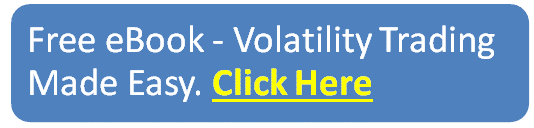blog

# What is Vomma: Everything You Need To Know

#### Options Trading 101 - The Ultimate Beginners Guide To Options

As Seen On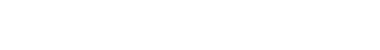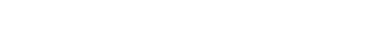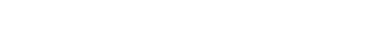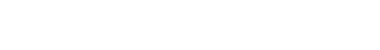by Gavin in Blog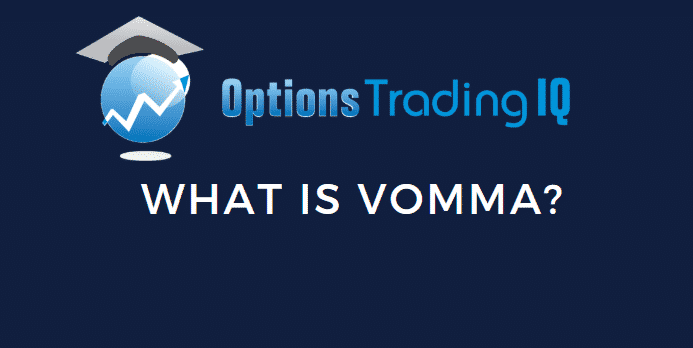What is vomma? Well, vomma is the second order sensitivity to volatility. It measures the rate of change to Vega as implied volatility changes.

## Introduction

Most traders are generally familiar with the standard option Greeks – Delta, Gamma, Theta, Vega and Rho.

Option Greeks are important for traders as they show the relationship between the price of an option to different factors, such as implied volatility or the price of the underlying.

The five Greeks mentioned above are called first-order greeks, as they can be computed based on an explicit formula based on specific factors.

There are however, another class of Greeks called the higher order Greeks.

These are based on changes to first-order Greeks, meaning that the higher order Greeks will tell you how another Greek will change.

They provide a useful tool for traders to be able to understand the dynamics of their portfolio exposure and spot opportunities called by extreme factors.

## What Is Vomma?

Vomma is one such example of a higher order Greek.

Sometimes called Volga, Vomma is simply a measurement of volatility, more specifically the second order sensitivity to volatility.

What this means, is that a trader can use Vomma to measure the rate of change to Vega as implied volatility changes.

Recall that Vega is a first-order Greek which shows the amount by which a call or put price will change, based on a one point change in implied volatility.Let’s work through an example, starting with Vega.

Say you had an option with a value of \$3.70 and a Vega of 10. This would mean that for every 1% increase in implied volatility, the option would rise by 10 cents.

Now we’ll introduce Vomma.

If an option has Vomma, it means that the Vega will change as implied volatility changes.

If the option had a normalised Vomma of 4, it means that when there is a 1% change in implied volatility, Vega will increase by 4.

Using our previous example, if there was a 1% change in implied volatility, Vega would go from 10 to 14. The value of the option would therefore likely increase by around 12 to \$3.82.

Why 12 and not 10 and 14?

Since Vega is changing as implied volatility changes, we can’t use the original Vega of 10 or the final Vega of 14.

Instead we need to take an average of the two numbers as an approximation of the average Vega value over the course of the implied volatility change.

## Vomma Across Different Strike Prices

Vomma will be highest for out-of-the-money options, while at-the-money options will have very low vomma.

This means that when implied volatility changes, the vega of the at-the-money options will not change as much as the out-of-the-money options.

Vega will remain fairly constant for at-the-money options.

Out-of-the-money options have high vomma because a rise in implied volatility makes it more likely that they could finish in-the-money.

## Conclusion

Since Vomma is used by traders to understand the rate of change to Vega as implied volatility changes, it serves as a useful tool for predicting price changes due to factors that aren’t included in standard options pricing models.

Option prices are calculated using the Black-Scholes options pricing model, but this model doesn’t include every possible factor that could affect an option.

By understanding the relationship between Vomma, Vega and option prices, traders can use it to exploit opportunities from temporary and extreme factors that are found outside of traditional options pricing models.